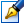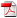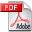##Integrability of cubic differential systems with invariant straight lines and invariant cubics

 Author: Dascalescu Anatoli Degree: doctor of Speciality: 01.01.02 - Differential equations Year: 2020 Scientific adviser: Dumitru Cozmadoctor habilitat, associate professor (docent) Institution: Institute of Mathematics and Computer Science

### Status

The thesis was presented on the 20 December, 2019
Approved by NCAA on the 28 February, 2020

### Abstract0.21 Mb / in romanian0.20 Mb / in english

### Thesis

CZU 517.9251.82 Mb / in romanian
152 pages

### Keywords

cubic differential system, invariant algebraic curve, the problem of the center, Darboux integrability, center sequence, the problem of cyclicity

### Summary

Thesis structure: introduction, four chapters, general conclusions and recommenda-tions, bibliography of 150 titles, 135 pages of main text. The obtained results were published in 15 scientific papers.

Domain of research: qualitative theory of dynamical systems, integrability of polyno¬mial differential systems.

Aim of the research: to determine the center conditions for the cubic differential system with two distinct invariant straight lines and one irreducible invariant cubic.

Objectives of the research: to obtain the conditions for the existence of two invariant straight lines and one irreducible invariant cubic for the cubic differential system with a singular point of a center or a focus; to study the integrability of the systems; to solve the problem of the center and the problem of cyclicity for the cubic systems with two invariant straight lines and one irreducible invariant cubic.

Novelty and scientific originality: the problem of the center, the problem of cyclicity and the problem of center sequences were solved for cubic differential systems with two invariant straight lines and one irreducible invariant cubic. It was proved that every cubic differential system with a center, having two invariant straight lines and one irreducible invariant cubic, is Darboux integrable or reversible.

The main scientific problem solved consists in establishing of some efficient relations between invariant algebraic curves, focus quantities and local integrability, which con¬tributed to the development of the Darboux integrability method. This made possible to obtain new sets of necessary and sufficient center conditions for cubic differential systems with two invariant straight lines and one invariant cubic.

The theoretical significance of the work: it was elaborated an efficient method in solving the problem of center based on relations between the existence of algebraic invariant curves, focus quantities and Darboux integrability.

The practical value of the work: the results obtained for cubic differential systems concerning the problem of the center and the problem of cyclicity represent an important step in solving the 16th Hilbert problem about limit cycles.

Implementation of the scientific results: the obtained results can be applied in investigations of the problem of integrability and the problem of limit cycles for polynomial differential systems; can serve as support for Master Thesis and some optional university courses for students and master students; can be used in the study of mathematical models which describe some social and natural processes.